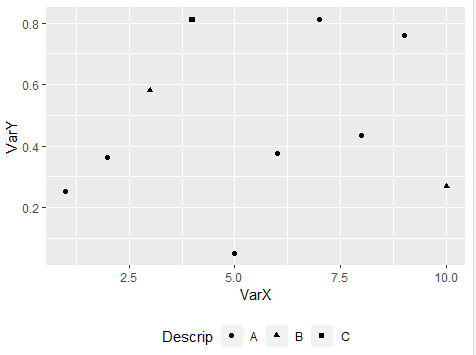2 views

I'm trying to create a ggplot2 plot with the legend beneath the plot.

The ggplot2 book says on p 112 "The position and justification of legends are controlled by the theme setting legend.position, and the value can be right, left, top, bottom, none (no legend), or a numeric position".

The following code works (since "right" it is the default), and it also works with "none" as the legend position, but "left", "top", "bottom", all fail with "Error in grid.Call.graphics("L_setviewport", pvp, TRUE) : Non-finite location and/or size for viewport"

library(ggplot2)

(myDat <- data.frame(cbind(VarX=10:1, VarY=runif(10)),

Descrip=sample(LETTERS[1:3], 10, replace=TRUE)))

qplot(VarX,VarY, data=myDat, shape=Descrip) +

opts(legend.position="right")

What am I doing wrong? Re-positioning a legend must be incredibly common, so I figure it's me.

by
edited

To move or position a legend in ggplot2, you can add a theme function to your plot as follows:

library(ggplot2)

(myDat <- data.frame(cbind(VarX=10:1, VarY=runif(10)),

Descrip=sample(LETTERS[1:3], 10, replace=TRUE)))

qplot(VarX,VarY, data=myDat, shape=Descrip) +

theme(legend.position = "bottom")If you want to explore more in R programming then watch this R programming tutorial for beginner: